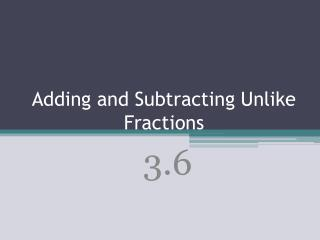Download Presentation# Adding and Subtracting Unlike Fractions - PowerPoint PPT PresentationDownload Presentation## Adding and Subtracting Unlike Fractions

- - - - - - - - - - - - - - - - - - - - - - - - - - - E N D - - - - - - - - - - - - - - - - - - - - - - - - - - -
##### Presentation Transcript

1. To add and subtract fractions with unlike denominators, first find a common denominator using one of these methods: Method 1 Find a common denominator by multiplying the two denominators. Method 2 Find the least common denominator(LCD) of the denominators. (Cake Method)

2. Steps • Find LCD or CD • Write equivalent fractions: Multiply the fraction by a value of 1 in order to get the LCD or CD • Add or subtract the numerators • Reduce

3. Write in Simplest form: • LCD: 18 • Rename Fractions: • Add the numerators:

4. Write the answer in simplest form. Find a common denominator: 4(5) = 20. Multiply by fractions equal to 1. + Rewrite with common denominators. Add numerators. Keep the denominator. Rewrite as a mixed number.

5. Write in Simplest Form:

6. Find the perimeter of the figure below. + First, work with the fractions. Then, work with the whole numbers. Last, simplify.

7. Vickie has cups of shredded cheese to put in her enchilada casserole and ¾ cup of cheese for the top. If she has 3 cups of cheese in all, how much cheese will she have left?

8. Homework: • Page 129 (14-46) even